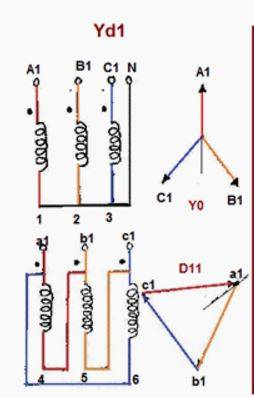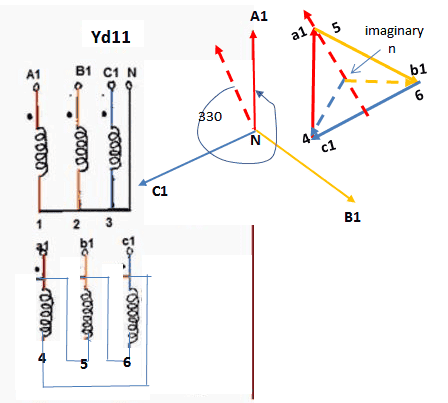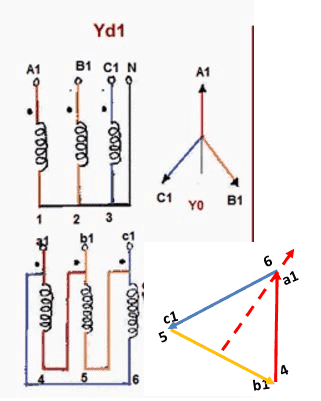# Help me understand transformer vector groups

Hi,

I have problems understanding why, for example, a Yd1 connection introduces a -30 degree phase shift, see image below.

How should I think when I want to produce vector groups like that, and derive myself what the phase shift should be?

Thanks..First - just consider the actual phase angle of C1-A1 relative to N-A1 - there will be a 30deg Shift there. So it is not really any magic in the transformer - each winding Primary and Secondary are aligned to the same phase angle.

If you keep the vectors properly aligned - you will see... for example in the bottom Delta configuration, c1-a1 vector should be vertical because it is in the same phase as N-A1 above.
Now using the same Vector angle for N-B1 and N-B2 - connect the "tail" of a1-b1 to the head of the c1-a1, and then the same for b1-c1 - this should close the triangle of the delta.

I proper transformer nameplate will maintain this same arrangement. EXAMPLE

•OliskaP
As windadct said A1_N is same phase as a1_4 , B1_N is same phase as b1_5, C1_N is same phase as c1_6 since the same magnetic flux will pass through both windings.
The connection will be as per attached sketch- for Yd11 : 5 with a1 ,6 with b1 and 4 with c1 and
for Yd1 a1_6, b1_4, c1_5.
The convention states an imaginary neutral point in the intersection of all three medians of the equilateral triangle , and an imaginary voltage to this neutral from each point of triangle. The a1 to neutral n is the first phase and the angle from HV A1_N is the considered angle which divided by 30 degrees gets the symbol of the connection[in Yd11 case 330/30=11].
You may find in IEC 60076-1 Annex D all the connections.•OliskaP
Very nice explanation, thanks, both of you.